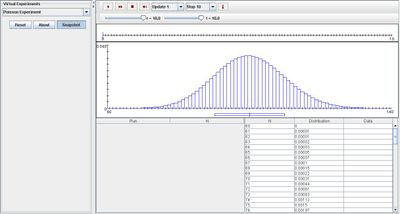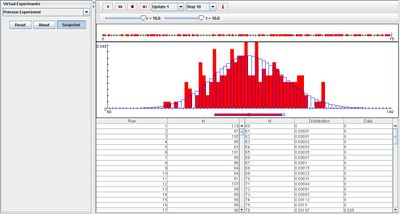# SOCR EduMaterials Activities TwoTypePoissonExperiment

## Description

The experiment is to run a Poisson process until time t. Each arrival is type 1 with probability p or type 0 with probability 1-p. In the timeline, the type 1 arrivals are shown as red dots and the type 0 arrivals as green dots. The number of type 1 arrivals M and the number of type 0 arrivals N are recorded on each update. The densities and moments of M and N are shown in the distribution graphs and the distribution tables. The parameters are the rate of the process r, the time t, and the probability p. These can be varied with the scroll bars above.

## Goal

To provide a simulation demonstrating each arrival in the Poisson process as two independent types that will assist others to develop a generalization of the Two-Type Poisson Experiment which is sometimes known as “Splitting” a Poisson process.

## Experiment

Go to the SOCR Experiment [] and select the Two - Type Poisson Experiment from the drop-down list of experiments on the top left. The image below shows the initial view of this experiment:When pressing the play button, one trial will be executed and recorded in the distribution table below. The fast forward button symbolizes the nth number of trials to be executed each time. The stop button ceases any activity and is helpful when the experimenter chooses “continuous,” indicating an infinite number of events. The fourth button will reset the entire experiment, deleting all previous information and data collected. The “update” scroll indicates nth number of trials (1, 10, 100, or 1000) performed when selecting the fast forward button and the “stop” scroll indicates the maximum number of trials in the experiment.

Because parameter t and r may be varied, both variables are able to modify the shape of the distribution graph. When either r or t increases, the distribution graph takes a more accurate shape of the normal curve, whereas decreasing the values of these two variables will cause the graph to be less normal. The images shown below demonstrate these effect as the first illustrates what happens when t is increased and the second illustrates what happens when both t and r are increased:As for parameter p, increasing it would make the right distribution graph more normal and the left distribution graph less normal. When it is decreased, the left distribution graph becomes more normal and the right distribution graph becomes less normal. This happens since both graphs represent M and N separately, where one displays the graph of 1-p and the other, p. The image below demonstrates this effect after a number of trials:Note that as the number of trials increase, the empirical density graph converges to the distribution graphs.

## Applications

The Two – Type Poisson Experiment is an applet that generalizes the importance of experiments when “splitting” a Poisson process. Each arrival in the Poisson process is one of two independent types. This experiment may be used in many different types of situations. For example,

Suppose that soda cans can either be underweight (type 1) or overlooked (type 0) by a counter.

Suppose students at the Student Store cold be typed as either male (type 1) or female (type 0).